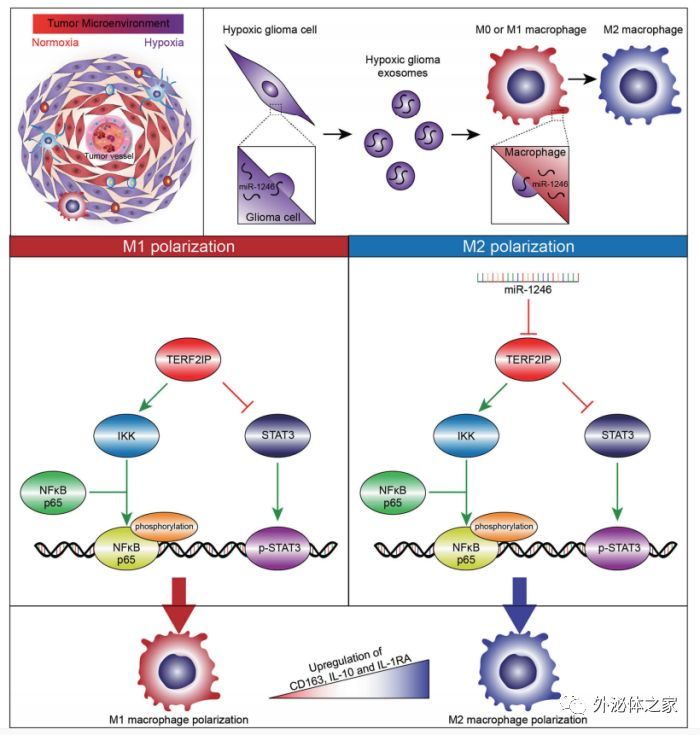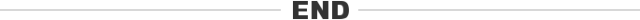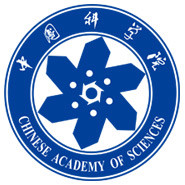## 山东大学齐鲁医院：缺氧胶质瘤衍生的外泌体传递miRNA-1246诱导M2巨噬细胞极化

9 月 9 日 外泌体之家```

```【科研成长加油站】0+0+

### 相关内容0+0+0+0+0+0+0+0+0+0+0+0+0+0+0+0+0+0+0+0+0+0+0+0+0+0+0+0+0+0+
Top